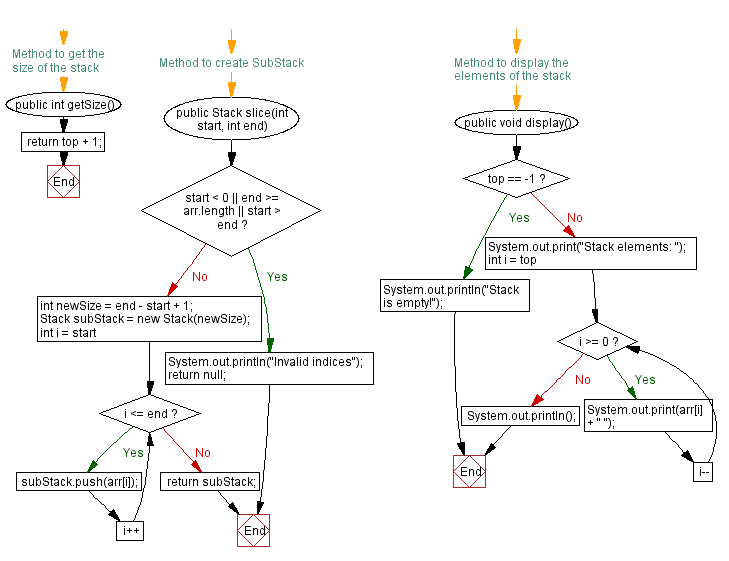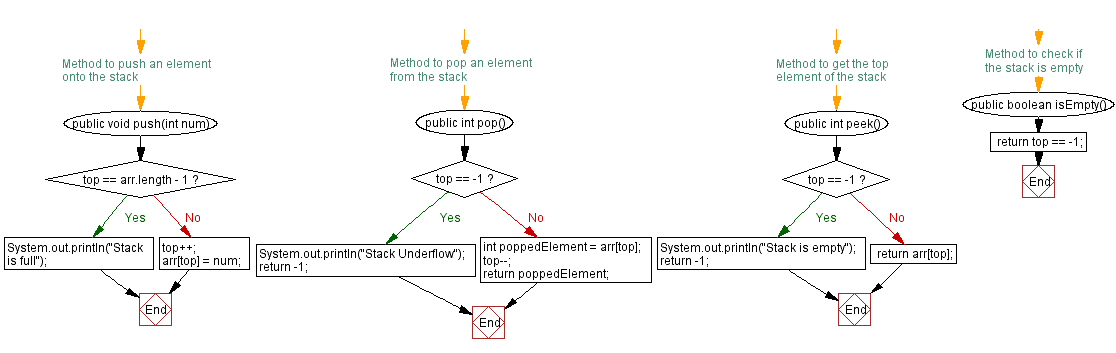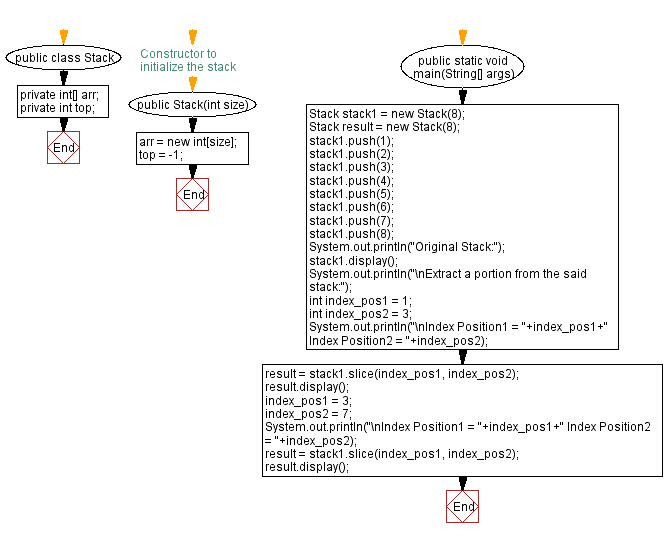# Java Exercises: New stack from a portion of the original stack

## Java Stack: Exercise-24 with Solution

Write a Java program that implements a stack and creates a new stack from a portion of the original stack.

Sample Solution:

Java Code:

``````import java.util.Scanner;

public class Stack {
private int[] arr;
private int top;

// Constructor to initialize the stack
public Stack(int size) {
arr = new int[size];
top = -1;
}

// Method to push an element onto the stack
public void push(int num) {
if (top == arr.length - 1) {
System.out.println("Stack is full");
} else {
top++;
arr[top] = num;
}
}

// Method to pop an element from the stack
public int pop() {
if (top == -1) {
System.out.println("Stack Underflow");
return -1;
} else {
int poppedElement = arr[top];
top--;
return poppedElement;
}
}

// Method to get the top element of the stack
public int peek() {
if (top == -1) {
System.out.println("Stack is empty");
return -1;
} else {
return arr[top];
}
}

// Method to check if the stack is empty
public boolean isEmpty() {
}

// Method to get the size of the stack
public int getSize() {
}

// Method to create SubStack
public Stack slice(int start, int end) {
if (start < 0 || end >= arr.length || start > end) {
System.out.println("Invalid indices");
return null;
}

int newSize = end - start + 1;
Stack subStack = new Stack(newSize);

for (int i = start; i <= end; i++) {
subStack.push(arr[i]);
}

return subStack;
}

// Method to display the elements of the stack
public void display() {
if (top == -1) {
System.out.println("Stack is empty!");
} else {
System.out.print("Stack elements: ");
for (int i = top; i >= 0; i--) {
System.out.print(arr[i] + " ");
}
System.out.println();
}
}

public static void main(String[] args) {

Stack stack1 = new Stack(8);
Stack result = new Stack(8);
stack1.push(1);
stack1.push(2);
stack1.push(3);
stack1.push(4);
stack1.push(5);
stack1.push(6);
stack1.push(7);
stack1.push(8);
System.out.println("Original Stack:");
stack1.display();
System.out.println("\nExtract a portion from the said stack:");
int index_pos1 = 1;
int index_pos2 = 3;
System.out.println("\nIndex Position1 = " + index_pos1 + " Index Position2 = " + index_pos2);
result = stack1.slice(index_pos1, index_pos2);
result.display();
index_pos1 = 3;
index_pos2 = 7;
System.out.println("\nIndex Position1 = " + index_pos1 + " Index Position2 = " + index_pos2);
result = stack1.slice(index_pos1, index_pos2);
result.display();
}
}
```
```

Sample Output:

```Original Stack:
Stack elements: 8 7 6 5 4 3 2 1

Extract a portion from the said stack:

Index Position1 = 1 Index Position2 = 3
Stack elements: 4 3 2

Index Position1 = 3 Index Position2 = 7
Stack elements: 8 7 6 5 4
```

Flowchart:Live Demo:

Java Code Editor:

Improve this sample solution and post your code through Disqus

Java Stack Previous: Elements from two stacks without duplicates.
Java Stack Exercises Next: Symmetric difference of two stacks.

What is the difficulty level of this exercise?

Test your Programming skills with w3resource's quiz.

﻿